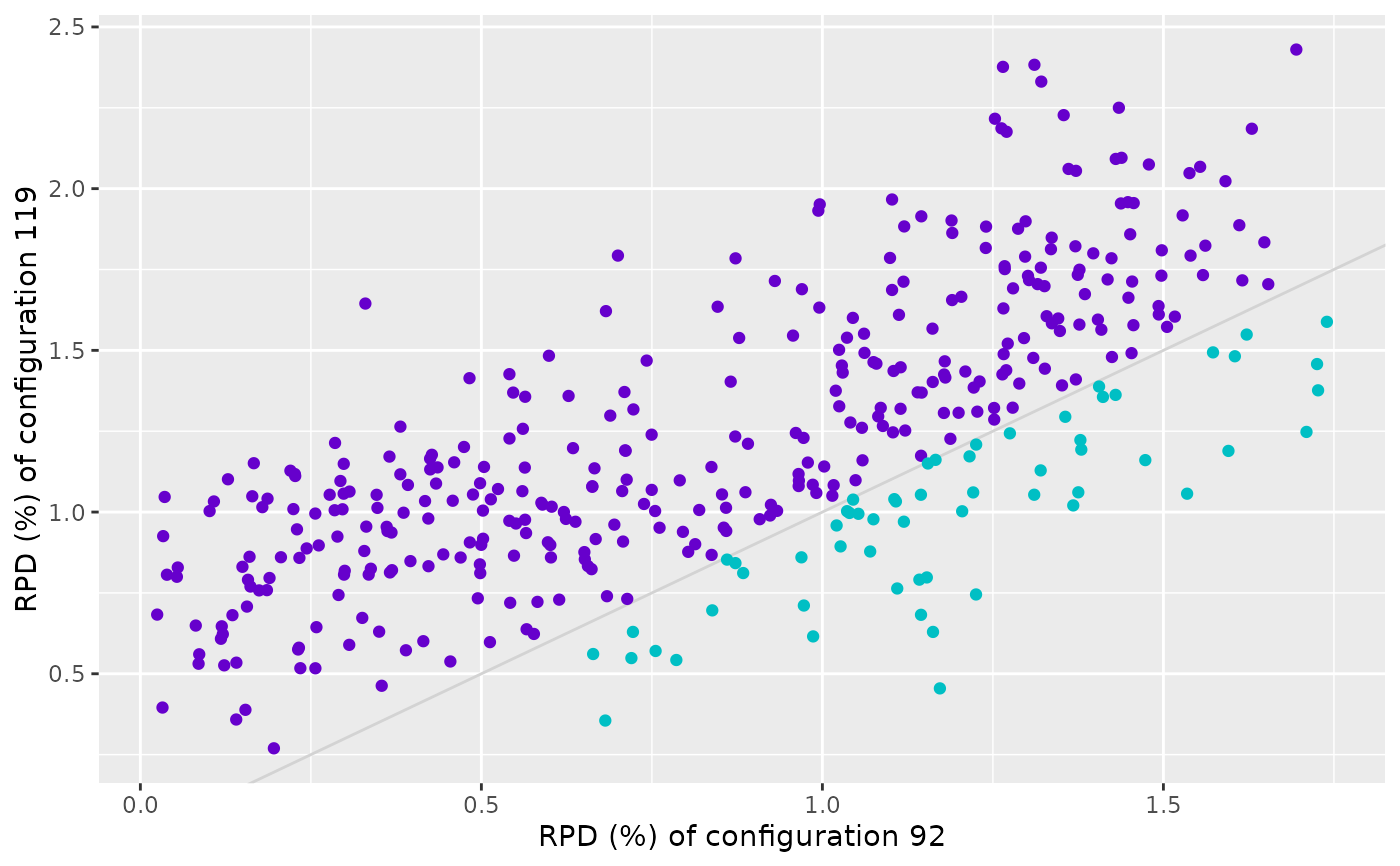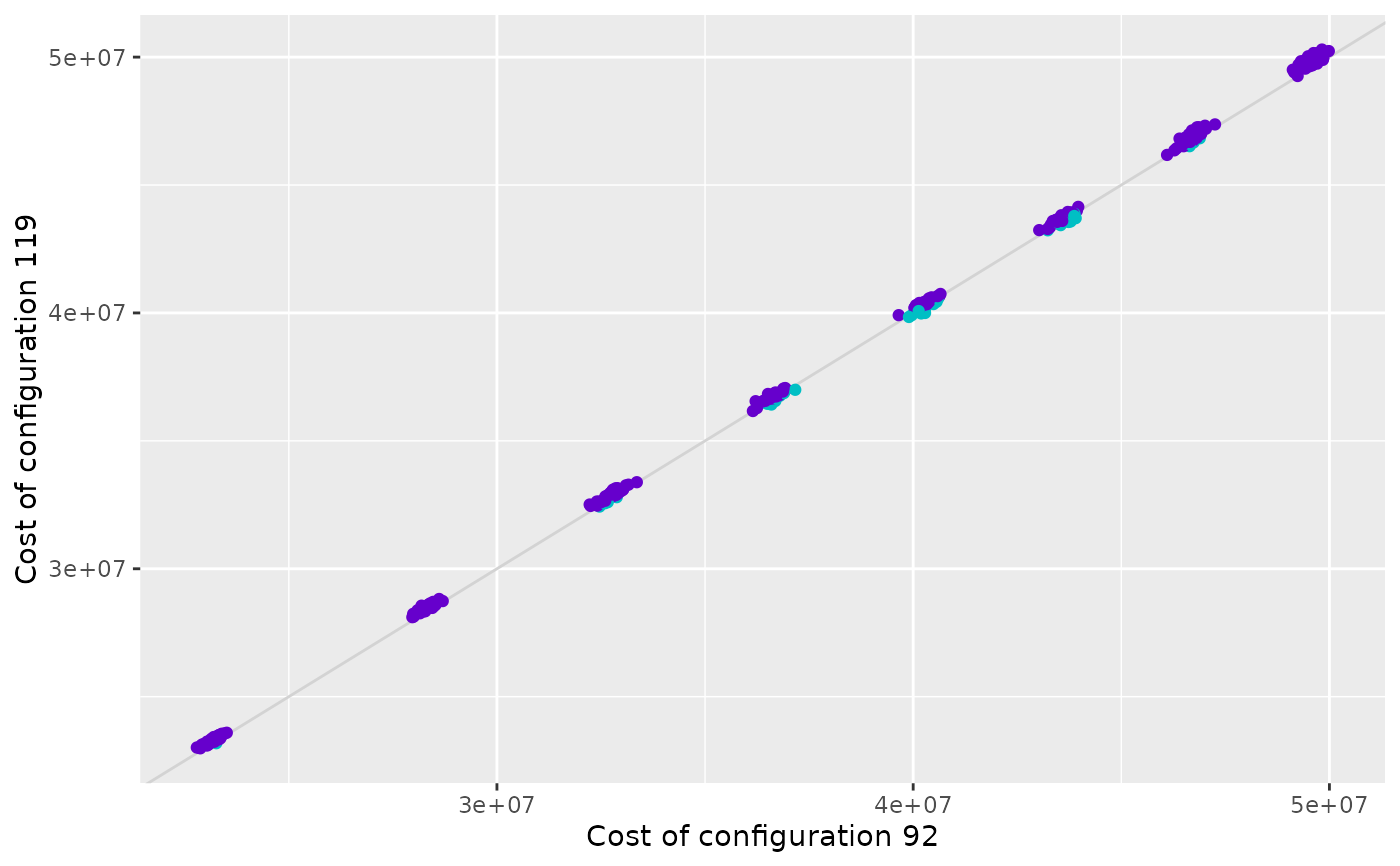Create a scatter plot that displays the performance of two configurations on a provided experiment matrix. Each point in the plot represents an instance and the color of the points indicates if one configuration is better than the other.

scatter_performance(
experiments,
x_id,
y_id,
rpd = TRUE,
filename = NULL,
interactive = base::interactive(),
instance_names = NULL
)

scatter_training(irace_results, ...)

scatter_test(irace_results, ...)

## Arguments

experiments

Experiment matrix obtained from irace training or testing data. Configurations in columns and instances in rows. As in irace, column names (configurations ids) should be characters. Row names will be used as instance names.

x_id, y_id

Configuration IDs for x-axis and y-axis, respectively.

rpd

(logical(1)) TRUE to plot performance as the relative percentage deviation to best results per instance, FALSE to plot raw performance.

filename

(character(1)) File name to save the plot, for example "~/path/example/filename.png".

interactive

(logical(1)) TRUE if the report may use interactive features (using plotly::ggplotly(), plotly::plot_ly() and DT::renderDataTable()) or FALSE if such features must be disabled. Defaults to the value returned by interactive(),

instance_names

Either a character vector of instance names in the same order as rownames(experiments) or a function that takes rownames(experiments) as input.

irace_results

The data generated when loading the .Rdata file created by irace (or the filename of that file).

...

Other arguments passed to scatter_performance().

## Value

ggplot2::ggplot() object

## Details

The performance matrix is assumed to be provided in the format of the irace experiment matrix thus, NA values are allowed. Consequently the number of evaluations can differ between configurations due to the elimination process applied by irace. This plot only shows performance data only for instances in which both configurations are executed.

scatter_training() compares the performance of two configurations on the training instances. The performance data is obtained from the evaluations performed by irace during the execution process.

scatter_test() compares the performance of two configurations on the test instances. The performance data is obtained from the test evaluations performed by irace. Note that testing is not enabled by default in irace and should be enabled in the scenario setup. Moreover, configuration ids provided in x_id and y_id should belong to elite configuration set evaluated in the test (see the irace package user guide for more details).

## Examples

iraceResults <- read_logfile(system.file(package="irace", "exdata",
"irace-acotsp.Rdata", mustWork = TRUE))
best_id <- iraceResults$iterationElites[length(iraceResults$iterationElites)]
scatter_performance(iraceResults\$experiments, x_id = 1, y_id = best_id)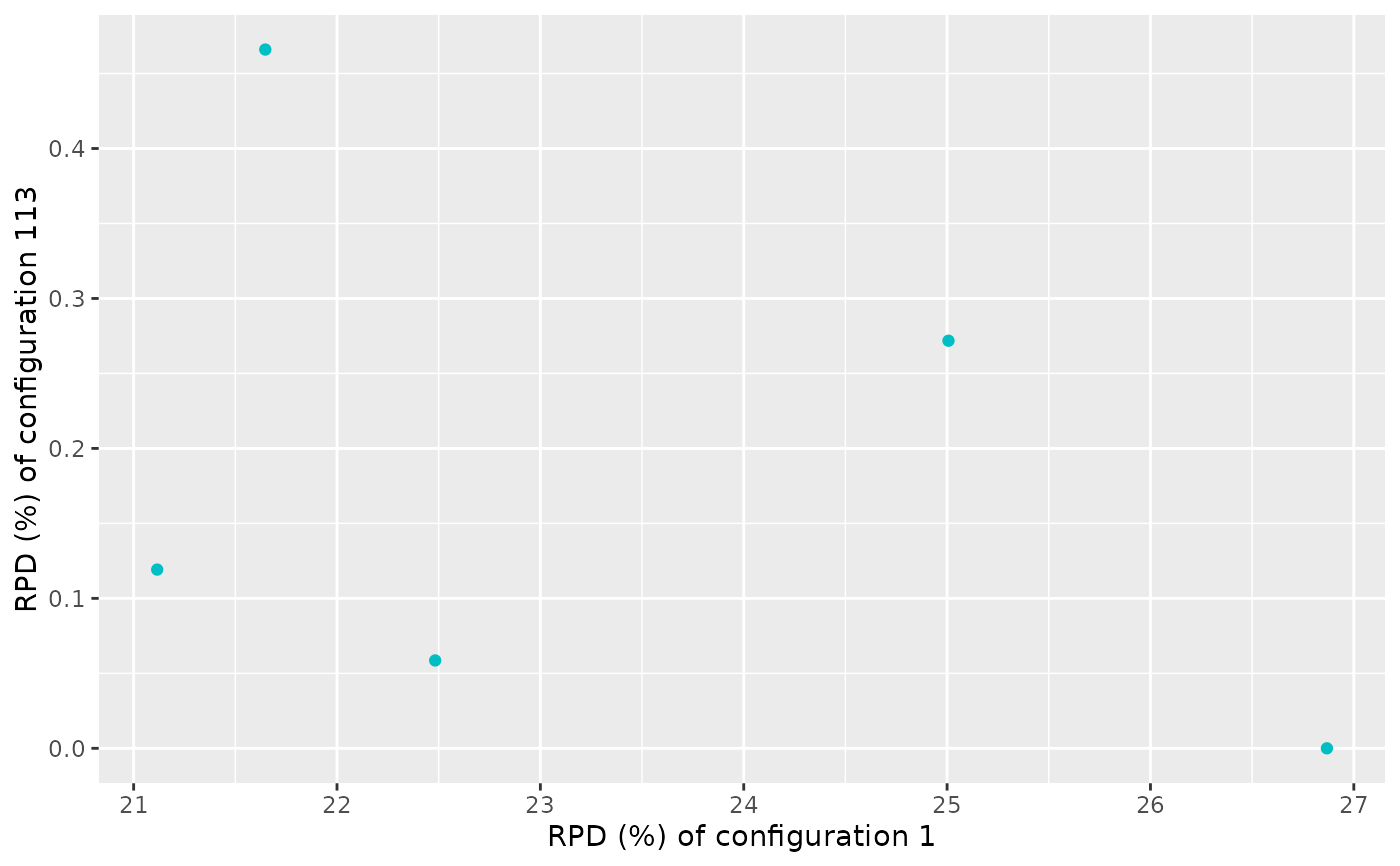"guide-example.Rdata", mustWork = TRUE))
scatter_training(iraceResults, x_id = 806, y_id = 809)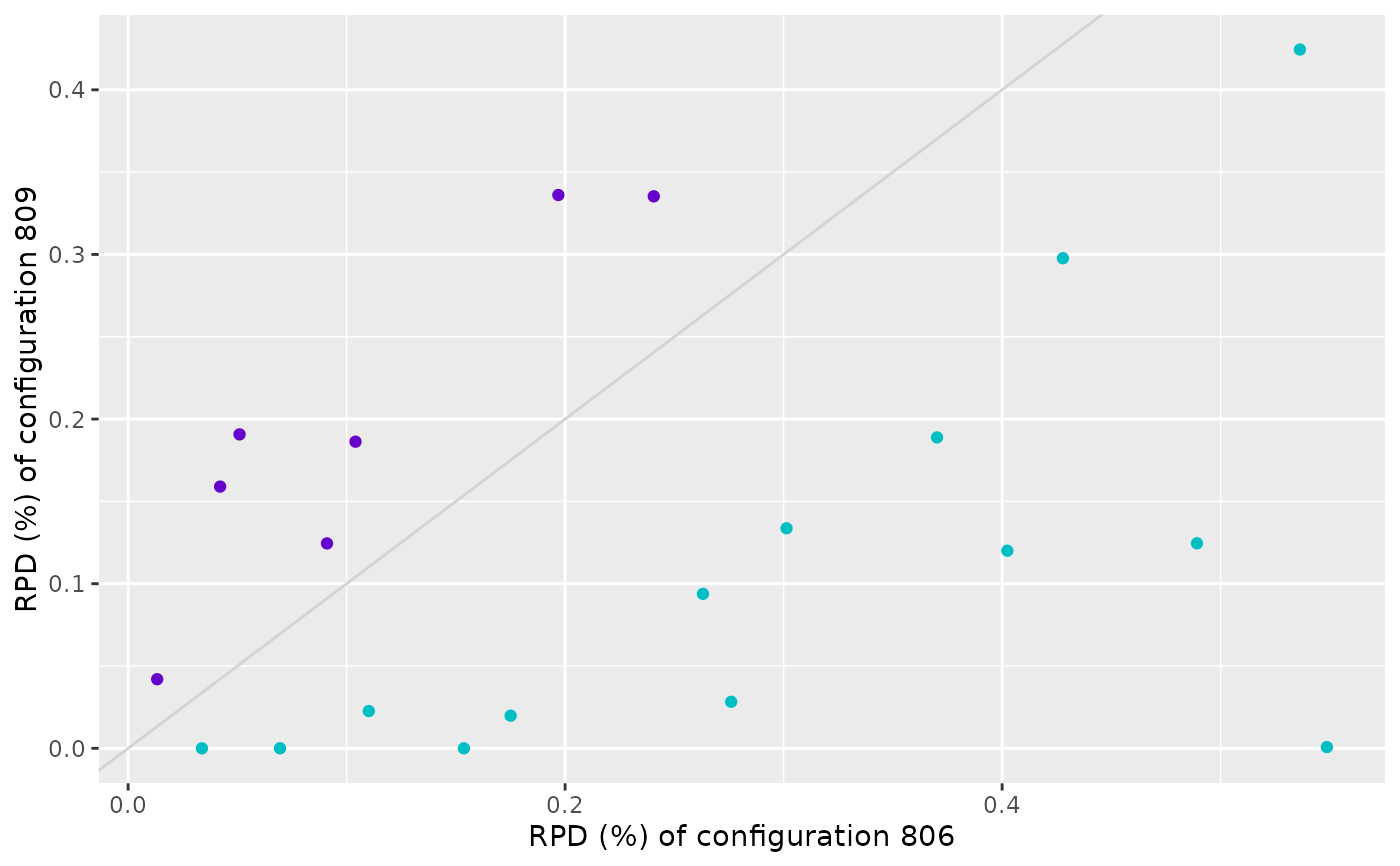# \donttest{
scatter_training(iraceResults, x_id = 806, y_id = 809, rpd = FALSE)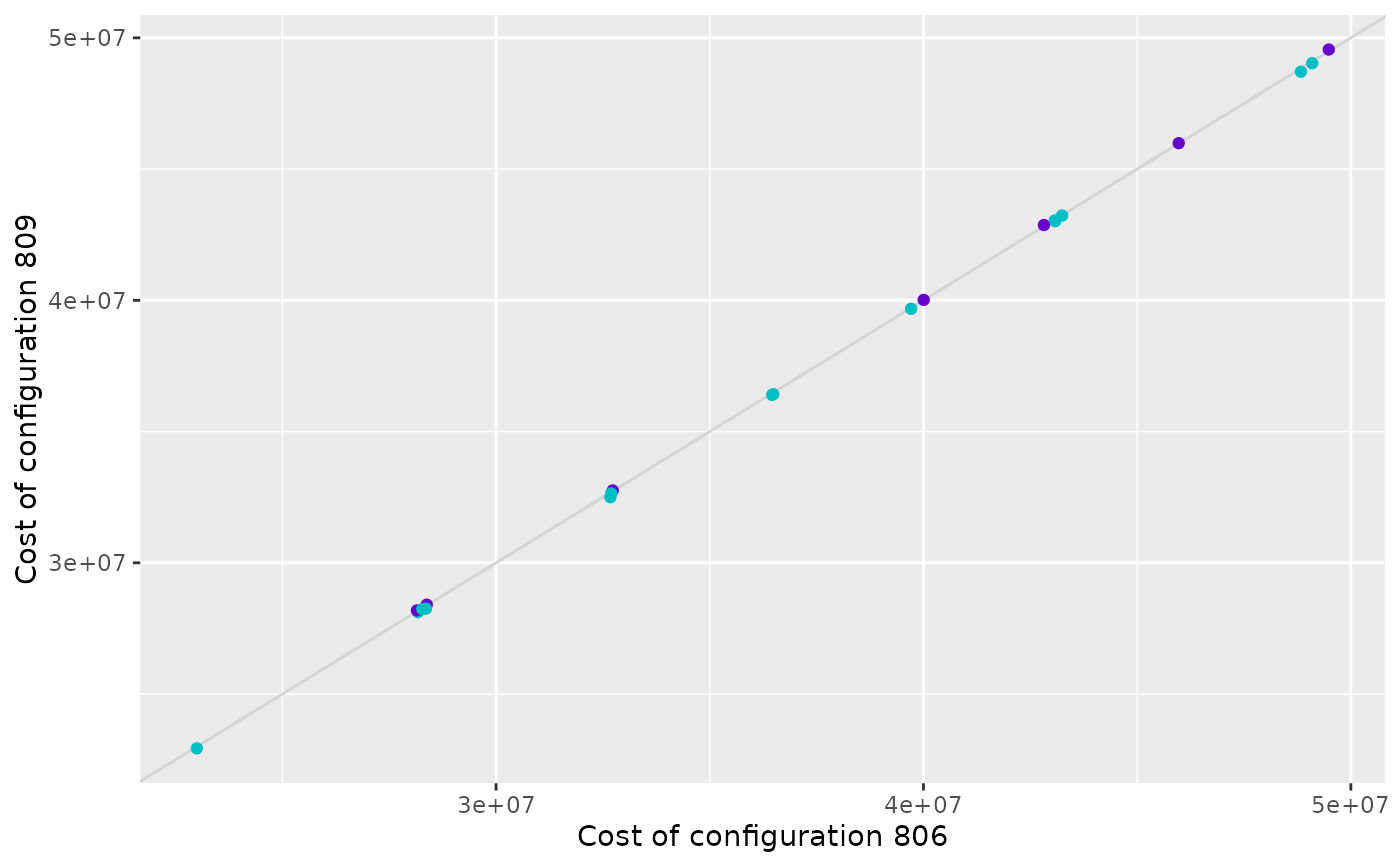# }